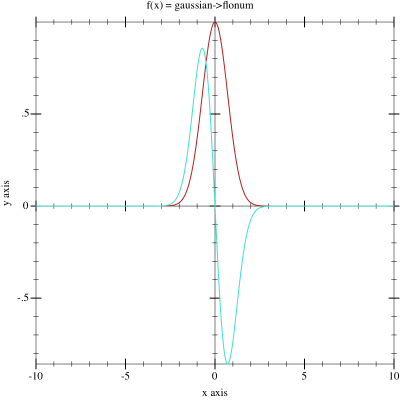7.8

### 2Module rml-neural/activation.

 (require rml-neural/activation) package: rml-neural

This module defines a set of activation functions, or method that may be used to determine the sensitivity of neurons in a network layer. To support both forward and backward propagation each method contains the activation function and it’s derivative. This Wikipedia page has a good overview of a number of activation functions.

#### 2.1Activation Function Structure

 value
 value
Contracts that encapsulate the pattern data-type or false.

 value
 value
Contracts used to define the procedures used in the structures below. Both activation and derivative functions are represented as a procedure that take a single, and return a single, real? or flonum?. They are equivalent to the following contract values.

 (-> real? real?) (-> flonum? flonum?)

See also Parallelism with Futures in The Racket Guide In general it is preferable to use the flonum-activator? structure and the corresponding flonum-activation/c form as this reduces the numeric conversions and allows optimization such as futures to work efficiently.

 struct(struct activator (name f df α)) name : symbol? f : real-activation/c df : real-activation/c α : maybe-real/c
This structure provides the activator function, it’s derivative, and an optional expectation value for a given method.

• f is the activation function, \phi(v_i)

• df is the activation derivative function, \phi^\prime(v_i) – sometimes shown as \phi^{-1}(v_i)

• α is an optional stochastic variable sampled from a uniform distribution at training time and fixed to the expectation value of the distribution at test time

 struct(struct flonum-activator activator (name f df α)) name : symbol? f : flonum-activation/c df : flonum-activation/c α : maybe-flonum/c
An extension to activator? that ensures that all values to the functions f and f as well as the value for α are guaranteed to be flonum?s. This allows for additional optimization and all math operations will be assumed to be flonum safe.

 procedure(make-activator name f df [α]) → activator? name : symbol? f : real-activation/c df : real-activation/c α : maybe-real/c = #f
 procedure(make-flonum-activator name f df [α]) → flonum-activator? name : symbol? f : flonum-activation/c df : flonum-activation/c α : maybe-flonum/c = #f
Construct an instance of activator? and flonum-activator? respectively. These constructors makes the value for α explicitly optional.

#### 2.2Activation Functions

Each of the activator? structures below will be defined by it’s activation function (the derivative is not shown). A sample plot shows the shape of the activation function in red and it’s derivative in turquoise.

 value
 value
\phi(v_i) = v_i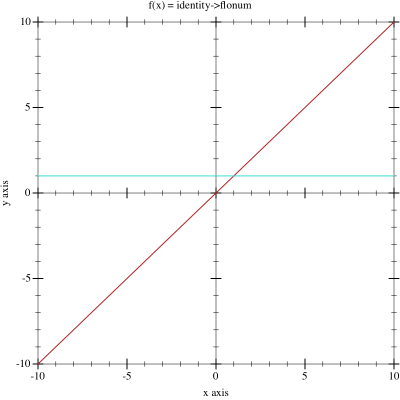value
 value
\phi(v_i) = \begin{cases} 0 & \text{for } v_i < 0\\ 1 & \text{for } v_i \geq 0 \end{cases}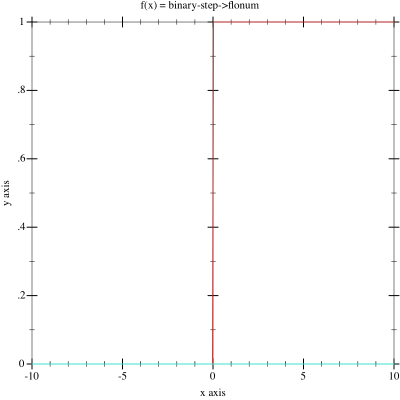value
 value
\phi(v_i) = \frac{1}{1+e^{-v_i}}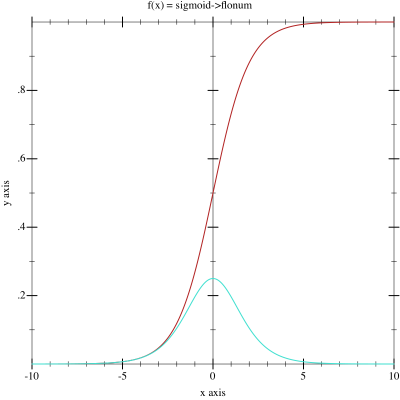value
 value
\phi(v_i) = \tanh(v_i)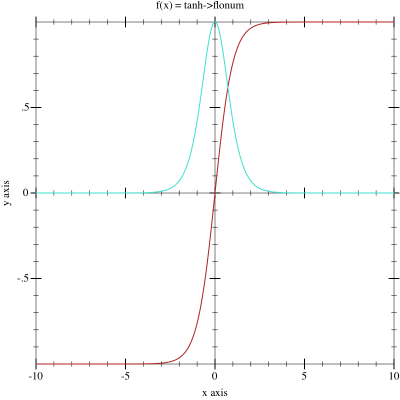value
 value
\phi(v_i) = \operatorname{atan}^{-1}(v_i)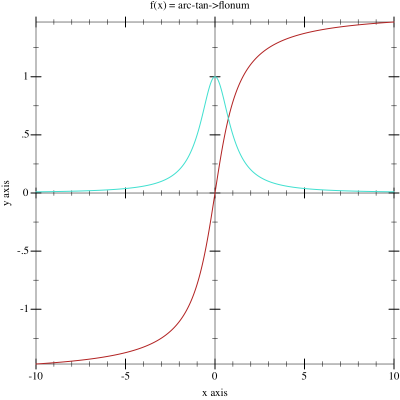value
 value
\phi(v_i) = \frac{v_i}{1+\left|v_i\right|}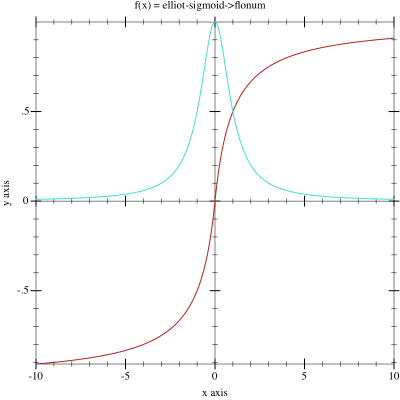procedure α : flonum?
 procedure α : number?
\phi(v_i) = \frac{v_i}{\sqrt{1+\alpha v_{i}^2}}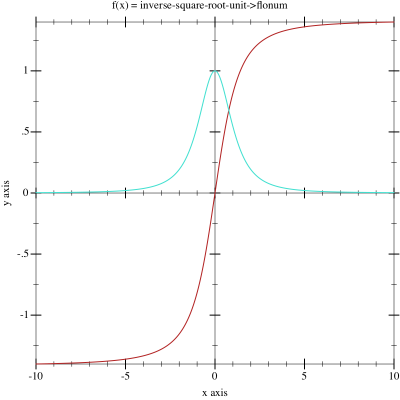procedure α : flonum?
 procedure α : number?
\phi(v_i) = \begin{cases} \frac{v_i}{\sqrt{1+\alpha v_{i}^2}} & \text{for } v_i < 0\\ v_i & \text{for } v_i \geq 0 \end{cases}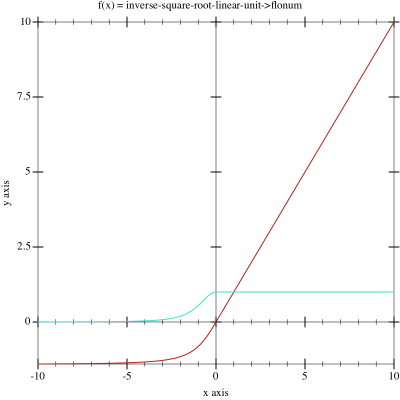value
 value
\phi(v_i) = \begin{cases} 0 & \text{for } v_i < 0\\ v_i & \text{for } v_i \geq 0 \end{cases}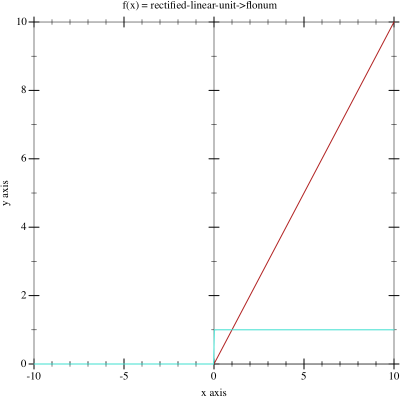procedure ∂ : flonum?
 value
 value
\phi(v_i) = \begin{cases} \delta v_i & \text{for } v_i < 0\\ v_i & \text{for } v_i \geq 0 \end{cases}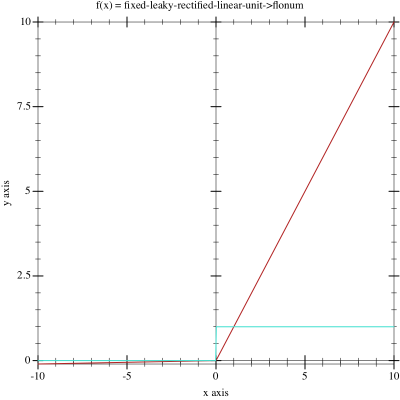Note that the fixed form of this activator uses a delta value \delta=0.01.

 value
 value
\phi(v_i) = \ln\left( 1 + e^{v_i} \right)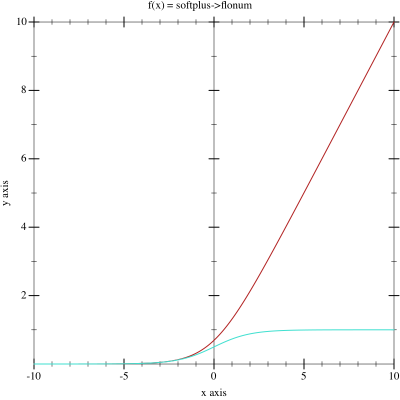value
 value
\phi(v_i) = \frac{\sqrt{v_{i}^2+1}-1}{2}+v_i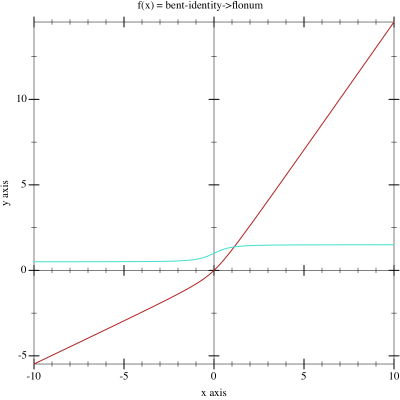value
 value
\phi(v_i) = \sin(v_i)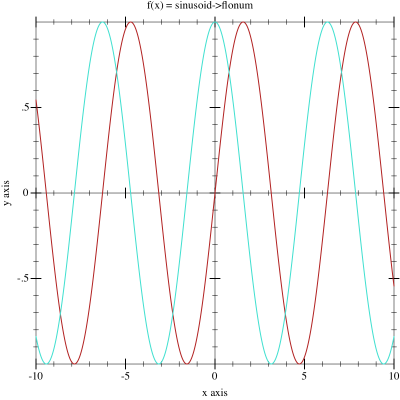value
 value
\phi(v_i) = \begin{cases} 1 & \text{for } v_i = 1\\ \frac{\sin(v_i)}{v_i} & \text{for } v_i \neq 0 \end{cases}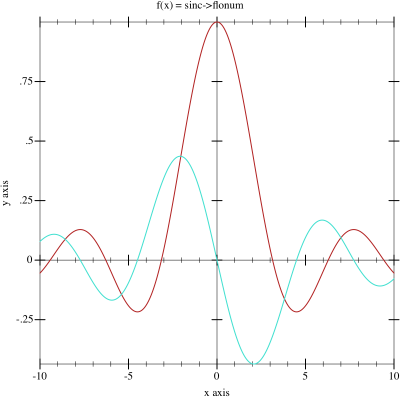value
 value
\phi(v_i) = e^{-v_{i}^2}